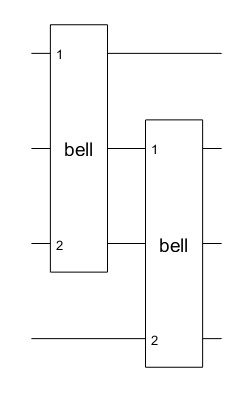# quantum.gate.CompositeGate class

Package: quantum.gate

Composite gate for quantum computing

Since R2023a

Installation Required: This functionality requires MATLAB Support Package for Quantum Computing.

## Description

A `CompositeGate` object contains a set of inner gates acting on a small set of qubits, and a mapping from this small set of qubits to the qubits of the circuit that contains the composite gate. The `CompositeGate` object fulfills the purpose of a subfunction in classical programming, where a set of inner gates can be packaged as a subcircuit to be used to construct an outer quantum circuit.

You can assign `CompositeGate` objects to the `Gates` property of a `quantumCircuit` object (as a vector of gates).

## Creation

Use the `compositeGate` creation function to construct a `CompositeGate` object.

You can also use the `qftGate` and `mcxGate` functions to construct `CompositeGate` objects. These functions construct specialized gates that applies the quantum Fourier transform and multi-controlled X gates, respectively.

## Properties

expand all

Name of the composite gate, specified as a string scalar. If you do not specify the name of the composite gate, the default value of this property is an empty string, `""`. Otherwise, the `Name` property value must start with a letter, followed by letters, digits, or underscores (with no white space).

When you construct a composite gate from an existing quantum circuit using the `compositeGate` function, the `Name` property of the circuit is copied to the `Name` property of the composite gate (unless you specify a new name when using `compositeGate`). This name is used in the plot of the composite gate and the function name in the generated QASM code.

Example: `"qft"`, `"bell"`, `"multi_controlled_Z"`

#### Attributes:

 `GetAccess` `public` `SetAccess` `public`

Control qubits of the composite gate, returned as empty. Creating a controlled composite gate is not supported and this property value is always empty.

#### Attributes:

 `GetAccess` `public` `SetAccess` `private`

Target qubits of the outer circuit containing the composite gate, returned as a numeric scalar or numeric vector of qubit indices. Each qubit of the inner gates in the `Gates` property is mapped to a qubit of an outer circuit containing the composite gate through the `TargetQubits` vector.

Example: `[3 4 7 8]`

#### Attributes:

 `GetAccess` `public` `SetAccess` `private`

Inner gates, returned as a column vector containing all the inner gates of the composite gate. The elements of this vector are of type `SimpleGate` or `CompositeGate`.

#### Attributes:

 `GetAccess` `public` `SetAccess` `private`

expand all

## Examples

collapse all

Create a quantum circuit that consists of Hadamard and controlled NOT gates to entangle two qubits. Name the circuit as `"bell"`.

```innerGates = [hGate(1); cxGate(1,2)]; innerCircuit = quantumCircuit(innerGates,Name="bell")```
```innerCircuit = quantumCircuit with properties: NumQubits: 2 Gates: [2×1 quantum.gate.SimpleGate] Name: "bell"```

Create an outer circuit that contains two composite gates constructed from this inner `"bell"` circuit. The first composite gate acts on qubits 1 and 3 of the outer circuit containing this gate. The second composite gate acts on qubits 2 and 4 of the outer circuit containing this gate.

```outerGates = [compositeGate(innerCircuit,[1 3]) compositeGate(innerCircuit,[2 4])]; outerCircuit = quantumCircuit(outerGates)```
```outerCircuit = quantumCircuit with properties: NumQubits: 4 Gates: [2×1 quantum.gate.CompositeGate] Name: ""```

Plot the outer circuit.

`plot(outerCircuit)`In a circuit diagram, each solid horizontal line represents a qubit. The top line is a qubit with index 1 and the remaining lines from top to bottom are labeled sequentially. In this example, the plotted outer circuit consists of four qubits with indices 1, 2, 3, and 4. The plot shows that qubits 1 and 3 of the outer circuit are mapped to qubits 1 and 2 of the inner circuit of the first composite gate, and qubits 2 and 4 of the outer circuit are mapped to qubits 1 and 2 of the inner circuit of the second composite gate.

Click one of the composite gate blocks in the plot. A new figure showing the internal gates of the composite gate appears.## Version History

Introduced in R2023a